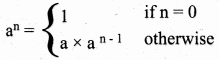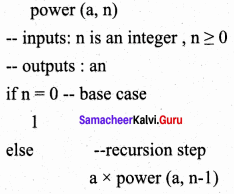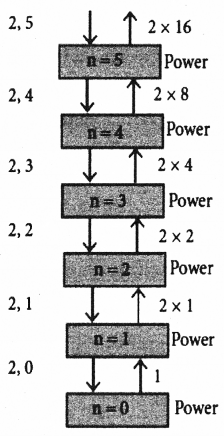Students can Download Computer Science Chapter 8 Iteration and Recursion Questions and Answers, Notes Pdf, Samacheer Kalvi 11th Computer Science Book Solutions Guide Pdf helps you to revise the complete Tamilnadu State Board New Syllabus and score more marks in your examinations.

## Tamilnadu Samacheer Kalvi 11th Computer Science Solutions Chapter 8 Iteration and Recursion

### Samacheer Kalvi 11th Computer Science Iteration and Recursion Text Book Back Questions and Answers

PART – 1

Question 1.
A loop invariant need not be true ………………….
(a) at the start of the loop
(b) at the start of each iteration
(c) at the end of each iteration
(d) at the start of the algorithm
(d) at the start of the algorithm

Question 2.
We wish to cover a chessboard with dominoes,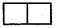the number of black squares and the number of white squares covered by dominoes, respectively, placing a domino can be modeled by ………………….
(a) b : = b + 2
(b) w : = w + 2
(c) b, w : = b + 1, w + 1
(d) b : = w
(c) b, w : = b + 1, w + 1

Question 3.
If m x a + n x b is an invariant for the assignment a, b : = a + 8, b + 7, the values of m and n are ………………….
(a) m = 8, n = 7
(b) m = 7, n = – 8
(c) m = 7, n = 8
(d) m = 8, n = – 7
(b) m = 7, n = – 8Question 4.
Which of the following is not an invariant of the assignment?
m, n : = m + 2, n + 3
(a) m mod 2
(b) n mod 3
(c) 3 x m – 2 x n
(d) 2 x m – 3 x n
(c) 3 x m – 2 x n

Question 5.
If Fibonacci number is defined recursively as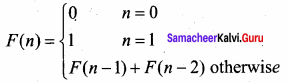to evaluate F(4), how many times F( ) is applied?
(a) 3
(b) 4
(c) 8
(d) 9
(d) 9

Question 6.
Using this recursive definition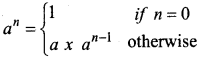how many multiplications are needed to calculate a10?
(a) 11
(b) 10
(c) 9
(d) 8
(b) 10

PART – 2

Question 1.
What is an invariant?
An expression involving variables, which remains unchanged by an assignment to one of these variables is called as an invariant of the assignment.

Question 2.
Define a loop invariant.
In iteration, the loop body is repeatedly executed as long as the loop condition is true. Each time the loop body is executed, the variables are updated. However, there is also a property of the variables which remains unchanged by the execution of the loop body. This unchanging property is called the loop invariant.Question 3.
Does testing the loop condition affect the loop invariant? Why?
No, the loop condition do not affect the loop invariant. Because the loop invariant is true at four points.

1. At the start of loop.
2. At the start of each iteration.
3. At the end of each iteration.
4. At the end of the loop.

Question 4.
What is the relationship between loop invariant, loop condition and the input – output recursively?
A loop invariant is a condition [among program variables] that is necessarily true immediately before and immediately after, each iteration of a loop. A loop invariant is some condition that holds for every iteration of the loop.

Question 5.
What is recursive problem solving?
Recursion is a method of solving problems that involves breaking a problem down into smaller and smaller sub problems until user gets in to a small problem that it can be solved trivially. Usually recursion involves a function calling itself. While it may not seem like much on the surface, recursion allows us to write elegant solutions to problems that may otherwise be very difficult to program.

Question 6.
Define factorial of a natural number recursively.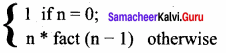Recursive Algorithm:
Fact (n)
– – inputs : n
– – outputs : Fact = n!
if (n = 0) – – base case
1
else
n * fact (n – 1) – – recursive step

PART – 3
III. Explain in Brief

Question 1.
There are 7 tumblers on a table, all standing upside down. You are allowed to turn any 2 tumblers simultaneously in one move. Is it possible to reach a situation when all the tumblers are right side up? (Hint: The parity of the number of upside down tumblers is invariant.)
Let u – No. of tumblers right side up
v – No. of tumblers up side down
Initial stage : u = 0, v = 7 (All tumblers upside down)
Final stage output: u = 7, v = 0 (All tumblers right side up)

Possible Iterations:
(i) Turning both up side down tumblers to right side up
u = u + 2, v = v – 2 [u is even]

(ii) Turning both right side up tumblers to upside down.
u = u – 2, v = v + 2 [u is even]

(iii) Turning one right side up tumblers to upside down and other tumbler from upside down to right side up.
u = u + 1 – 1 = u, v = v + 1 – 1 = v [u is even]

Initially u = 0 and continuous to be even in all the three cases. Therefore u is always even. Invariant: u is even (i. e. No. of right side up tumblers are always even)
But in the final stage (Goal), u = 7 and v = 0 i. e. u is odd.
Therefore it is not possible to reach a situation where all the tumblers are right side up.Question 2.
A knockout tournament is a series of games. Two players complete in each game; the loser is knocked out (i.e. does not play any more), the winner carries on. The winner of the tournament is the player that is left after all other players have been knocked out. Suppose there are 1234 players in a tournament. How many games are played before the tournament winner is decided?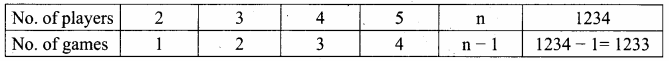On the other hand let n be the number of games, played and r be the number of players remaining in the tournament.
After every game, r will be reduced by 1.
r → no. of players remaining
n → no. of games played
If r = 2 then n = 1
As n increases, r decreases
n, r : = n + 1, r – 1
n + r = (n + 1) + (r – 1)
= n + 1 + r – 1
= n + r
Therefore n + r is invariant. n + r = 1234 (No. of players initially)
The winner of the tournament is the player that is left after all other players have been knocked out.
After all the games, only one player (winner) is left out.
i. e. n = 1
Put n = 1 in (1)
n + r = 1234 …. (1)
1 + r = 1234
r = 1234 – 1 = 1233
No. of games played = 1233

Question 3.
King Vikramaditya has two magic swords. With one, he can cut off 19 heads of a dragon, but after that the dragon grows 13 heads. With the other sword, he can cut off 7 heads, but 22 new heads grow. If all heads are cut off, the dragon dies. If the dragon has originally 1000 heads, can it ever die? (Hint: The number of heads mod 3 is invariant.)
No. of heads of dragon = 1000
Let n be the number of heads of the dragon at initial state.

Case 1 : King uses Sword 1
n : = n – 19 + 13 = n – 6 No. of heads are reduced by 6.

Case 2 : King uses Sword 2
n : = n – 7 + 22 = n + 15
No. of heads are increased by 15.

Note:
In the above two cases either 6 heads are removed or 15 heads added. Both 6 and 15 are multiples of 3.
Therefore repeating case 1 and case 2 recursively will either reduce or increase dragon heads in multiples of 3.
That is the invariant is n mod 3.
If n mod 3 = 0 then there is a possibility that the dragon dies.
But 1000 is not a multiple of 3 1000 mod 3 = 1 ≠ 0
It is not possible to kill the dragon. The dragon never dies.

PART – 4
IV. Explain in Detail

Question 1.
Assume an 8 x 8 chessboard with the usual coloring. “Recoloring” operation changes the color of all squares of a row or a column. You can recolor repeatedly. The goal is to attain just one black square. Show that you cannot achieve the goal. (Hint: If a row or column has b black squares, it changes by (|(8 – b) – b|).
In a chess board no. of squares in any row or column = 8
Total No. of squares = 8 x 8 = 64
No. of black squares = 32
No. of white squares = 32
Let No. of blacks in a selected recoloring row or column = b
No. of black squares after recoloring operation = 8 – b
Initial state b = 32
Desired final state b = 1
Let us tabulate all the possible outcomes after recoloring a row or column.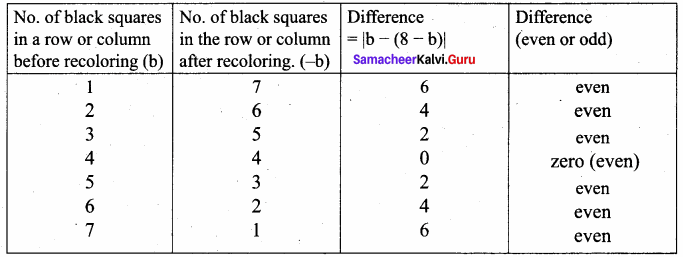Difference is the no. of black squares left out in a row or column after recoloring operation. Difference is even. Difference is invariant.
But our goal is one ( 1 is odd) black square, so, it is not possible to attain the goal.

Question 2.
Power can also be defined recursively as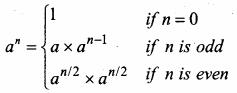Construct a recursive algorithm using this definition. How many multiplications are needed to calculate a10?
Power:
power (5, 2) = 5 x 5 = 25
power (x, n) raise x to the power n

Algorithm: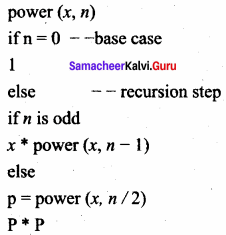To find a10: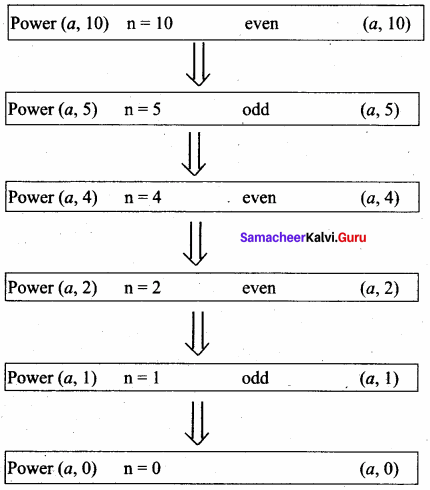Question 3.
A single – square – covered board is a board of 2n x 2n squares in which one square is covered with a single square tile. Show that it is possible to cover this board with triominoes without overlap.
size of the board is 2nn x 2n
Number of squares = 2n x 2n = 4n
Number of squares covered = 1
Number of squares to be covered = 4n – 1
4n – 1 is a multiple of 3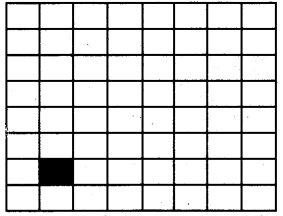Case 1 : n = 1
The size of the board 2 x 2
one triominoe can cover 3 squares without overlap.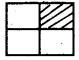We can cover it with one triominoe and solve the problem.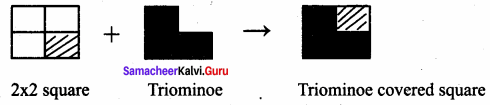Case 2 : n ≥ 2
1. place a triominoe at the center of the entire board so as to not cover the covered sub – board.

2. One square in the board is covered by a tile. The board has 4 sub – boards of size 22n – 1 x 22n – 1.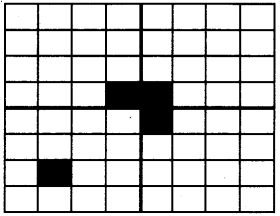Out of 4 sub – boards one sub – board is a single square covered sub – board.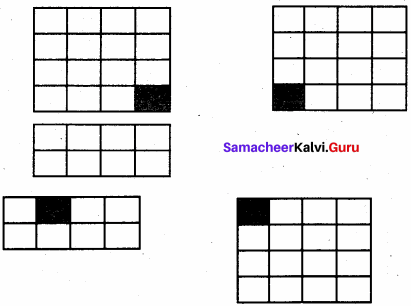One triominoe can cover remaining three sub – boards into single square covered sub – board. The problem of size n is divided into 4 sub – problems of size (n – 1). Each sub – board has 22n – 1 x 22n – 1 – 1 = 22n – 2 – 1 = 4n – 1 – 1 squares to be covered.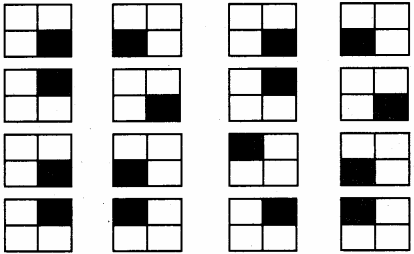4n – 1 – 1 is also a multiple of 3
In this, the 2n x 2n board is reduced to boards of size 2×2 having are square covered. A triominoe can be placed in each of these boards and hence the whole original 2n x 2n . board is covered with triominoe with out overlap.

### Samacheer kalvi 11th Computer Science Iteration and Recursion Additional Questions and Answers

PART – 1

Question 1.
………………… is an algorithm design technique, closely related to induction.
(a) Iteration
(b) Invariant
(c) Loop invariant
(d) Recursion
(d) Recursion

Question 2.
In which year E W Dijkstra was awarded ACM Turing Award?
(a) 1972
(b) 1974
(c) 1970
(d) 1911
(a) 1972Question 3.
In a loop, if L is an invariant of the loop body B, then L is known as a …………………
(a) recursion
(b) variant
(c) loop invariant
(d) algorithm
(c) loop invariant

Question 4.
Recursion must have at least ………………… base case.
(a) one
(b) two
(c) three
(d) four
(a) one

Question 5.
The unchanged variables of the loop body is …………………
(a) loop invariant
(b) loop variant
(c) condition
(d) loop variable
(a) loop invariant

Question 6.
………………… is the algorithm design techniques to execute the same action repeatedly.
(a) Iteration
(b) Recursion
(c) Both a & b
(d) none of these
(c) Both a & b

Question 7.
If L is a loop variant, then it should be true at ………………… important points in the algorithm.
(a) 2
(b) 3
(c) 4
(d) 5
(c) 4

Question 8.
The loop invariant need not be true at the …………………
(a) Start of the loop
(b) end of the loop
(c) end of each iteration
(d) middle of algorithm
(d) middle of algorithm

Question 9.
In an expression if the variables has the same value before and after an assignment, then it is of an assignment.
(a) variant
(b) Invariant
(c) iteration
(d) variable
(b) Invariant

Question 10.
The input size to a sub problem is than the input size to the original problem.
(a) equal
(b) smaller
(c) greater
(d) no criteria
(b) smaller

Question 11.
When the solver calls a sub solver, then it is called …………………
(a) Iterative call
(b) solver call
(c) recursive call
(d) conditional call
(c) recursive call

Question 12.
How many cases are needed for a recursive solvers?
(a) 2
(b) 3
(c) 4
(d) 5
(a) 2Question 13.
Which of the following is updated when each time the loop body is executed?
(a) data type
(b) subprogram
(c) function
(d) variable
(d) variable

Question 14.
Which is the key to constract iterative algorithm …………………
(a) loop invariant
(b) Variable
(c) loop
(d) Recursive
(a) loop invariant

PART – 3

Question 1.
When the loop variant will be true?
The loop invariant is true before the loop body and after the loop body, each time.

Question 2.
What is an invariant?
An expression involving variables, which remains unchanged by an assignment to one of these variables, is called an invariant of the assignment.

PART – 3
III. Explain in Brief

Question 1.
Write a note on Recursion.
Recursion:
Recursion is another algorithm design technique, closely related to iteration, but more powerful. Using recursion, we solve a problem with a given input, by solving the same problem with a part of the input, and constructing a solution to the original problem from the solution to the partial input.

Question 2.
Write down the steps to be taken to construct a loop.

1. Establish the loop invariant at the start of the loop.
2. The loop body should so update the variables as to progress toward the end, and maintain the loop invariant, at the same time.
3. When the loop ends, the termination condition and the loop invariant should establish the input – output relation.Question 3.
Write a note on Iteration.
Iteration:
In iteration, the loop body is repeatedly executed as long as the loop condition is true. Each time the loop body is executed, the variables are updated. However, there is also a property of the variables which remains unchanged by the execution of the loop body. This unchanging property is called the loop invariant. Loop invariant is the key to construct and to reason about iterative algorithms.

PART – 4
IV. Explain in Detail

Question 1.
Explain Loop invariant with a near diagram.
In a loop, if L is an invariant of the loop body B, then L is known as a loop invariant, while C
– – L
B
– – L
The loop invariant is true before the loop body and after the loop body, each time. Since L is true at the start of the first iteration, L is true at the start of the loop also (just before the loop). Since L is true at the end of the last iteration, L is true when the loop ends also (just after the loop). Thus, if L is a loop variant, then it is true at four important points in the algorithm, as annotated in the algorithm.

1. At the start of the loop (just before the loop)
2. at the start of each iteration (before loop body)
3. at the end of each iteration (after loop body)
4. at the end of the loop (just after the loop)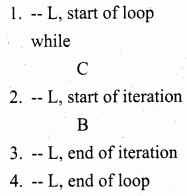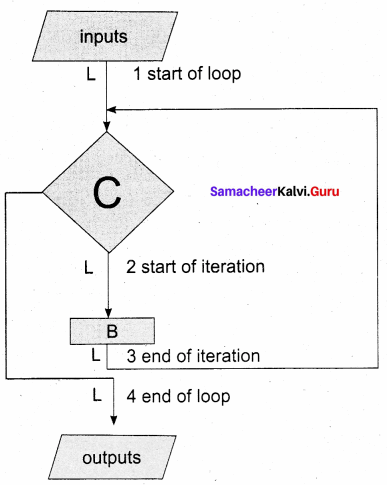Question 2.
Explain recursive problem solving.
To solve a problem recursively, the solver reduces the problem to sub – problems, and calls another instance of the solver, known as sub – solver, to solve the sub – problem. The input size to a sub – problem is smaller than the input size to the original problem. When the solver calls a sub – solver, it is known as recursive call. The magic of recursion allows the solver to assume that the sub – solver (recursive call) outputs the solution to the sub – problem. Then, from the solution to the sub – problem, the solver constructs the solution to the given problem. As the sub – solvers go on reducing the problem into sub – problems of smaller sizes, eventually the sub-problem becomes small enough to be solved directly, without recursion. Therefore, a recursive solver has two cases:
1. Base case:
The problem size is small enough to be solved directly. Output the solution. here must be at least one base case.

2. Recursion step:
The problem size is not small enough. Deconstruct the problem into a sub – problem, strictly smaller in size than the given problem. Call a sub – solver to solve the sub problem. Assume that the sub – solver outputs the solution to the sub problem. Construct the solution to the given problem. This outline of recursive problem solving technique is shown below.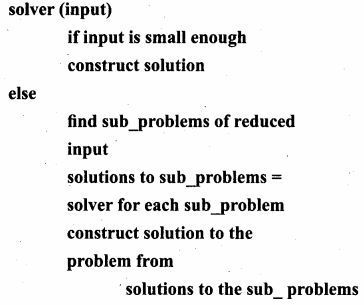Whenever we solve a problem using recursion, we have to ensure these two cases: In the recursion step, the size of the input to the recursive call is strictly smaller than the size of the given input, and there is at least one base case.

Question 3.
Give an example for loop invariant.
The loop invariant is true in four crucial points in a loop. Using the loop invariant, we can construct the loop and reason about the properties of the variables at these points.
Example:
Design an iterative algorithm to compute an. Let us name the algorithm power(a, n). For example,
power(10, 4) = 10000
power (5, 3) = 125
power (2, 5) = 32
Algorithm power(a, n) computes an by multiplying a cumulatively n times,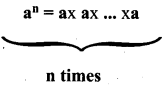The specification and the loop invariant are shown as comments.
power (a, n)
– – inputs: n is a positive integer
– – outputs: p = an
p, i : = 1, 0
while i ≠ n
– – loop invariant: p = ai
p, i : = p x a, i + 1
The step by step execution of power (2, 5) is shown in Table. Each row shows the values of the two variables p and i at the end of an iteration, and how they are calculated. We see that p = ai is true at the start of the loop, and remains true in each row. Therefore, it is a loop invariant.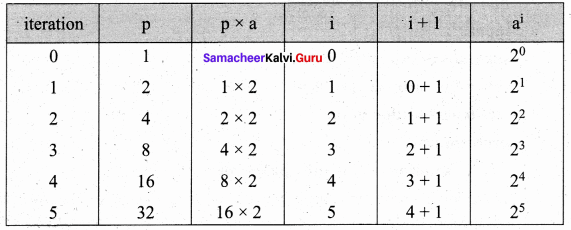When the loop ends, p = ai is still true, but i = 5. Therefore, p = a5. In general, when the loop ends, p = an . Thus, we have verified that power(a, n) satisfies its specification.

Question 4.
There are 6 equally spaced trees and 6 sparrows sitting on these trees,one sparrow on each tree. If a sparrow flies from one tree to another, then at the same time, another sparrow flies from its tree to some other tree the same distance away, but in the opposite direction. Is it possible for all the sparrows to gather on one tree?
Let us index the trees from 1 to 6. The index of a sparrow is the index of the tree it is currently sitting on. A pair of sparrows flying can be modeled as an iterative step of a loop. When a sparrow at tree i flies to tree i + d, another sparrow at tree j flies to tree j – d. Thus, after each iterative step, the sum S of the indices of the sparrows remains invariant. Moreover, a loop invariant is true at the start and at the end of the loop.
At the start of the loop, the value of the invariant is
S = 1 + 2 + 3 + 4 + 5 + 6 = 21
When the loop ends, the loop invariant has the same value. However, when the loop ends, if all the sparrows were on the same tree, say k, then S = 6k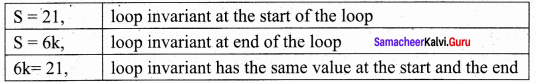It is not possible – 21 is not a multiple of 6. The desired final values of the sparrow indices is not possible with the loop invariant. Therefore, all the sparrows cannot gather on one tree.

Question 5.
Customers are waiting in a line at a counter. The man at the counter wants to know how many customers are waiting in the line.
Customers are waiting in a line at a counter. The man at the counter wants to know how many customers are waiting in the line.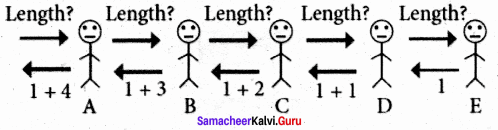Instead of counting the length himself, he asks customer A for the length of the line with him at the head, customer A asks customer B for the length of the line with customer B at the head, and so on. When the query reaches the last customer in the line, E, since there is no one behind him, he replies 1 to D who asked him. D replies 1 + 1 = 2 to C, C replies 1 + 2 = 3 to B, B replies 1 + 3 = 4 to A, and A replies 1 + 4 = 5 to the man in the counter.

Question 6.
Design a recursive algorithm to compute an. We constructed an iterative algorithm to compute an in Example 8.5. an can be defined recursively as